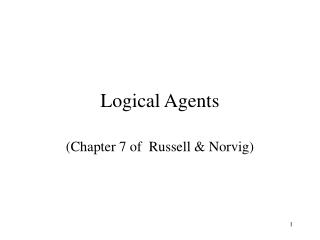DownloadDownload PresentationLogical Agents

Logical Agents

Télécharger la présentationLogical Agents

- - - - - - - - - - - - - - - - - - - - - - - - - - - E N D - - - - - - - - - - - - - - - - - - - - - - - - - - -
Presentation Transcript

1. Logical Agents (Chapter 7 of Russell & Norvig)

2. Outline • Knowledge bases • Logic • Propositional (Boolean) Logic • Normal forms • Inference Rules

3. Knowledge bases • knowledge base = set of sentences in a formal language • Declarative approach to building KB • Query approach to extracting knowledge

4. Wumpus Example

5. Knowledge based agent • Represent states, actions • Incorporate new percepts & update internal representations • Deduce hidden properties & appropriate actions

6. Knowledge based agents function KB-AGENT(percept) returns an actionstatic:KB, a knowledge base t, a counter, initially 0, indicating time TELL(KB, MAKE-PERCEPT-SENTENCE(percept, t)) action <- ASK(KB, MAKE-ACTION-QUERY(t)) TELL(KB, MAKE-ACTION-SENTENCE(action, t)) t <- t + 1 returnaction

7. Logics • Formal language • for knowledge representation • drawing conclusions • Syntax • defines language structure • Semantics • defines language meaning

8. Types of Logic • Characterised by primitives • Ontological, Epistemological

9. Entailment • KB |= alpha • A knowledge base entails a sentence alpha • if and only if alpha • is true in all worlds where KB is true

10. Inference • KB |-ialpha = sentence alpha can be derived from KB by procedure i • Soundness of i • whenever KB |-ialpha then KB |= alpha • Completeness of i • whenever KB |= alpha then KB |-ialpha

11. Propositional logic: Syntax • simplest logic • S is a sentence, then so is NOT(S) • S1 and S2 sentences, then so is • S1 AND S2 • S1 OR S2 • S1 => S2 • S1 <=> S2

12. Propositional logic: Semantics • NOT(S) is true iff S is false • S1 AND S2 is true iff S1 is true and S2 is true • S1 OR S2 is true iff S1 is true or S2 is true • S1 => S2 is true iff S1 is false or S2 is true • I.e is false iff S1 is true and S2 is false • S1 <=> S2 is true iff S1 => S2 is true and • S2 => S1 is true

13. Propositional inference: Enumeration method • alpha = A OR B • KB = (A OR C) AND (B OR not C) • Does KB |= alpha ? • To prove check all possibilities • Enumerate the table

14. Normal forms • Inference via Syntatic operations on normal forms • Conjunctive Normal Form (CNF) • conjunction of disjunctions • Disjunctive Normal Form (DNF) • disjunction of conjunctions • Horn Form • conjunction of Horn clauses

15. Validity • A sentence is valid if it is true in all models • true for all possible values of variables • e.g. A OR not A • A => A • (A AND (A => B)) => B • Validity related to inference • KB |= alpha if and only if (KB => alpha ) is valid

16. Satisfiability • A sentence is satisfiable if true in some model • that is true for one set of values • e.g. A OR B • C • A sentence is unsatisfiable if not true in any model • no set of values to make it true • e.g. A AND not A

17. Satisfiability and Inference • KB |= alpha if and only if (KB AND not alpha ) is unsatisfiable • This is called reductio ad absurdum • proof by contradiction

18. Proof methods • Model Checking • truth table enumeration • sound and complete • NP-complete problem • Application of inference rules • Generation of new sentences from old • Proof:- a sequence of inference rule applications • use inference rule as operators in search algorithm

19. Inference Rules:propositional logic • Resolution (for CNF) • alpha OR beta, (not beta) OR gamma • ------------------------------------------- • alpha OR gamma • Complete for propositional logic • Modus Ponens ( complete for Horn form) • alpha1,.. alphaN , alpha1AND..alphaN => beta • ------------------------------------------- • beta

20. Summary • Logical agents • apply inference to knowledge base • Basic concepts • syntax & semantics • entailment • inference • soundness • completeness